## Friction Questions and Answers Part-9

1. A block of mass m lying on a rough horizontal plane is acted upon by a horizontal force P and another force Q inclined at an angle $\theta$ to the vertical. The block will remain in equilibrium, if the coefficient of friction between it and the surface isa) $\frac{\left(P+Q\sin\theta\right)}{\left(mg+Q\cos\theta\right)}$
b) $\frac{\left(P\cos\theta+Q\right)}{\left(mg-Q\sin\theta\right)}$
c) $\frac{\left(P+Q\cos\theta\right)}{\left(mg+Q\sin\theta\right)}$
d) $\frac{\left(P\cos\theta-Q\right)}{\left(mg-Q\sin\theta\right)}$

Explanation:2. Which of the following is correct, when a person walks on a rough surface
a) The frictional force exerted by the surface keeps him moving
b) The force which the man exerts on the floor keeps him moving
c) The reaction of the force which the man exerts on floor keeps him moving
d) None of the above

Explanation: The reaction of the force which the man exerts on floor keeps him moving

3. A block of mass 0.1 kg is held against a wall by applying a horizontal force of 5 N on the block. If the coefficient of friction between the block and the wall is 0.5, the magnitude of the frictional force acting on the block is
a) 2.5 N
b) 0.98 N
c) 4.9 N
d) 0.49 N

Explanation:4.A body of mass M is kept on a rough horizontal surface (friction coefficient $\mu$ ). A person is trying to pull the body by applying a horizontal force but the body is not moving. The force by the surface on the body is F, where
a) $F= Mg$
b) $F=\mu Mgf$
c) $Mg\leq F\leq Mg\sqrt{1+\mu^{2}}$
d) $Mg\geq F\geq Mg\sqrt{1+\mu^{2}}$

Explanation: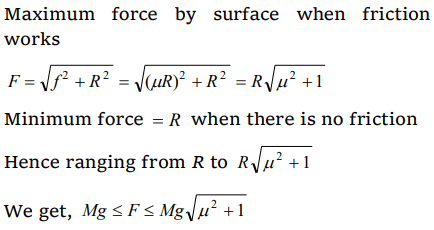5. What is the maximum value of the force F such that the block shown in the arrangement, does not move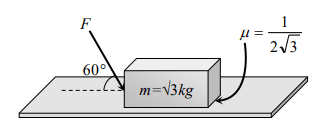a) 20 N
b) 10 N
c) 12 N
d) 15 N

Explanation:F = 20N

6. A block P of mass m is placed on a frictionless horizontal surface. Another block Q of same mass is kept on P and connected to the wall with the help of a spring of spring constant k as shown in the figure. $\mu_{s}$ is the coefficient of friction between P and Q. The blocks move together performing SHM of amplitude A. The maximum value of the friction force between P and Q isa) kA
b) $\frac{kA}{2}$
c) Zero
d) $\mu_{s}mg$

Explanation: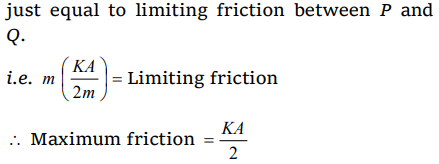7. A body of mass m rests on horizontal surface. The coefficient of friction between the body and the surface is $\mu$ . If the mass is pulled by a force P as shown in the figure, the limiting friction between body and surface will be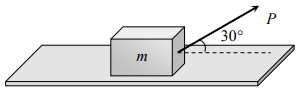a) $\mu mg$
b) $\mu \left[mg+\left(\frac{P}{2}\right)\right]$
c) $\mu \left[mg-\left(\frac{P}{2}\right)\right]$
d) $\mu \left[mg-\left(\frac{\sqrt{3}P}{2}\right)\right]$

Explanation: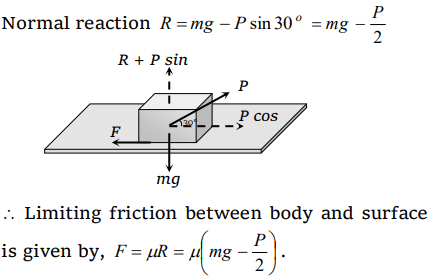8.A 40 kg slab rests on a frictionless floor as shown in the figure. A 10 kg block rests on the top of the slab. The static coefficient of friction between the block and slab is 0.60 while the kinetic friction is 0.40. The 10 kg block is acted upon by a horizontal force 100 N. If$g=9.8m\diagup s^{2}$ , the resulting acceleration of the slab will be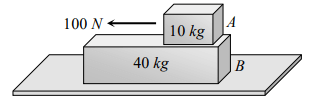a) $0.98m\diagup s^{2}$
b) $1.47m\diagup s^{2}$
c) $1.52m\diagup s^{2}$
d) $6.1m\diagup s^{2}$

Explanation: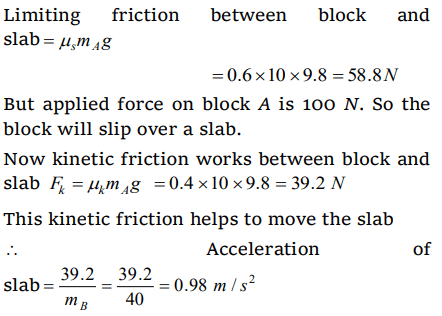9. A block of mass 2 kg rests on a rough inclined plane making an angle of 30° with the horizontal. The coefficient of static friction between the block and the plane is 0.7. The frictional force on the block is
a) 9.8 N
b) $0.7\times 9.8\times\sqrt{3}N$
c) $9.8\times\sqrt{3}N$
d) $0.8\times 9.8 N$